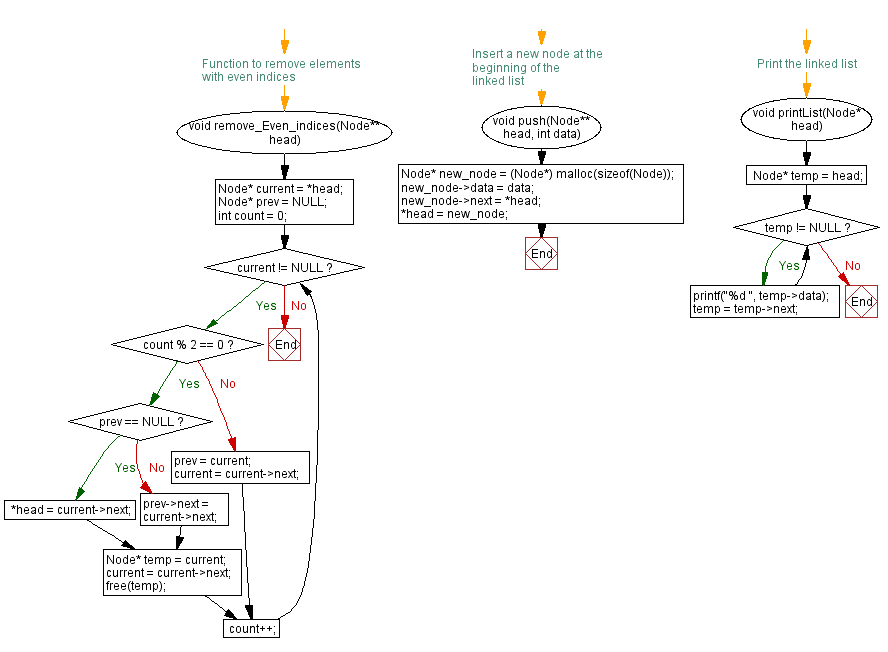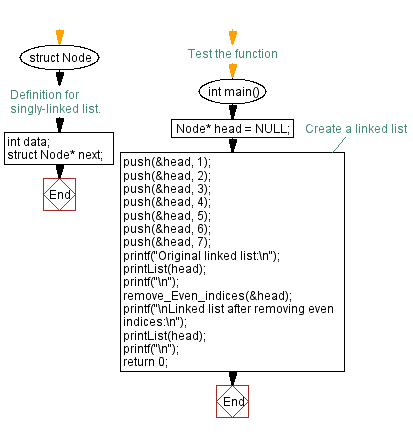﻿ C Program: Remove elements with even indices from a linked list

# C Exercises: Remove elements with even indices from a linked list

## C Singly Linked List : Exercise-26 with Solution

Write a C program that removes elements with even indices from a singly linked list.

Sample Solution:

C Code:

``````#include<stdio.h>
#include <stdlib.h>

struct Node {
int data;
struct Node* next;
};

// Function to remove elements with even indices
Node* prev = NULL;
int count = 0;

while (current != NULL) {
if (count % 2 == 0) {
if (prev == NULL) {
} else {
prev->next = current->next;
}
Node* temp = current;
current = current->next;
free(temp);
} else {
prev = current;
current = current->next;
}
count++;
}
}

// Insert a new node at the beginning of the linked list
void push(Node** head, int data) {
Node* new_node = (Node*) malloc(sizeof(Node));
new_node->data = data;
}

while (temp != NULL) {
printf("%d ", temp->data);
temp = temp->next;
}
}

// Test the function
int main() {

printf("\n");

printf("\nLinked list after removing even indices:\n");
printf("\n");

return 0;
}
```
```

Sample Output:

```Original linked list:
7 6 5 4 3 2 1

Linked list after removing even indices:
6 4 2
```

Flowchart :C Programming Code Editor:

What is the difficulty level of this exercise?

﻿